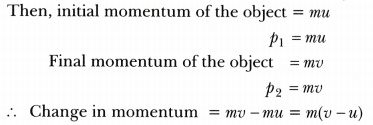# Derive the mathematical relation of Newton’s second law of motion

Derive the mathematical relation of Newton’s second law of motion